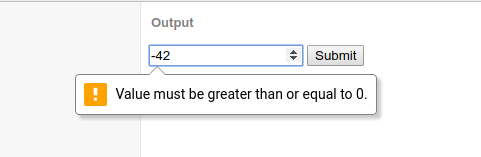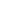| lesson material | material summary | questions and answers | definitions | types and examples | other information | materi pelajaran | ringkasan materi | pertanyaan dan jawaban | definisi | jenis-jenis dan contoh-contoh | informasi lainnya |

# HTML Tutorial: How to Limit Maximum and Minimum Input Values ​​on FormAttributes `min`and `max`attributes are to limit the maximum and minimum values ​​that can be entered on the form. This attribute is usually used in input related to numbers.
For example, you cannot input negative numbers, numbers more than 10, dates less than years `2000`, etc.
Whereas for input in the form of text, this attribute cannot work.
Let's try directly with the example:

## Negative Numbers cannot be input on Form

In order not to input the negative, then we need to limit the minimum value to zero ( `0`).
``````<input type="number" min="0" />
``````
The code above will produce:
Try entering a negative number by clicking on the downward icon, the result will be fixed in the value `0`.
But…
We can still give negative values ​​by typing directly. How come?
Yep, that can indeed be done. But when we submit HTML 5 it will tell you that the input value is wrong.Let's try another example.

## Only Accept Numbers from 1 to 5

Values ​​that can be inputted from 1 to 5, then we only need to determine the smallest value 1 and the biggest 5.
``````<input type="number" min="1" max="5" />
``````
The code above will produce:

## Only Can Enter Date above Year 2000

In order for the specified date above 2000, we need to set the lower limit (min) to be `2000-12-30`.
``````<input type="date" min="2000-12-30" />
``````
The code above will produce:

## The final word…

From some of the examples above, we can understand the functions of attributes `min`and `max`Then it's up to you what you want to apply.
##### 0 Komentar untuk "HTML Tutorial: How to Limit Maximum and Minimum Input Values ​​on Form"

Silahkan berkomentar sesuai artikel

Template By Kunci Dunia